The clock select algorithm determines from a set of sources, which are correct (truechimers) and which are not (falsetickers) according to a set of formal correctness assertions. The principles are based on the observation that the maximum error in determining the offset of a candidate cannot exceed one-half the roundtrip delay to the primary reference clock at the time of measurement. This must be increased by the maximum error that can accumulate since then. The selection metric, called the root distance,, is one-half the roundtrip root delay plus the root dispersion plus minor error contributions not considered here.

First, a number of sanity checks is performed to sift the selectable candidate from among the source population. The sanity checks are summarized as follows:

1. A stratum error occurs if (1) the source had never been synchronized or (2) the stratum of the source is below the `floor` option or not below the `ceiling` option of the `tos` command. The default values for these options are 0 and 15, respectively. Note that 15 is a valid stratum, but a server operating at that stratum cannot synchronize clients.

2. A distance error occurs for a source if the root distance (also known as synchronization distance) of the source is not below the distance threshold `maxdist` option of the `tos` command. The default value for this option is 1.5 s for networks including only the Earth, but this should be increased to 2.5 s for networks including the Moon.

3. A loop error occurs if the source is synchronized to the client. This can occur if two peers are configured with each other in symmetric modes.

4. An unreachable error occurs if the source is unreachable or if the `server` or `peer` command for the source includes the `noselect` option.

Sources showing one or more of these errors are considered nonselectable; only the selectable candidates are considered in the following algorithm. Given the measured offset θ0 and root distance λ, this defines a correctness interval0 − λ, θ0 + λ] of points where the true value of θ lies somewhere on the interval. The given problem is to determine from a set of correctness intervals, which represent truechimers and which represent falsetickers. The principles must be given a precise definition. The intersection interval is the smallest interval containing points from the largest number of correctness intervals. An algorithm that finds the intersection interval was devised by Keith Marzullo in his doctoral dissertation. It was first implemented in the Digital Time Synchronization Service (DTSS) for the VMS operating system for the VAX.

While the NTP algorithm is based on DTSS, it remains to establish which point in the correctness interval represents the best estimate of the offset for each candidate. The best point is at the midpoint θ0 of the correctness interval; however, the midpoint might not be within the intersection interval. A candidate with a correctness interval that contains points in the intersection interval is a truechimer and the best offset estimate is the midpoint of its correctness interval. A candidate with a correctness interval that contains no points in the intersection interval is a falseticker.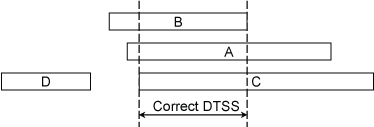Figure 1. Intersection Interval

Figure 1 shows correctness intervals for each of four candidates A, B, C and D. We need to find the maximum number of candidates that contain points in common. The result is the interval labeled DTSS. In the figure there are three truechimers A, B and C, and one falseticker D. In DTSS any point in the intersection interval can represent the true time; however, as shown below, this may throw away valuable statistical information. In any case, the clock is considered correct if the number of truechimers found in this way are greater than half the total number of candidates.

The question remains, which is the best point to represent the true time of each correctness interval? Fortunately, we already have the maximum likelihood estimate at the midpoint of each correctness interval. But, while the midpoint of candidate C is outside the intersection interval, its correctness interval contains points in common with the intersection interval, so the candidate is a truechimer and the midpoint is chosen to represent its time.

The DTSS correctness assertions do not consider how best to represent the truechimer time. To support the midpoint choice, consider the selection algorithm as a method to reject correctness intervals that cannot contribute to the final outcome; that is, they are falsetickers. The remaining correctness intervals can contribute to the final outcome; that is, they are truechimers. Samples in the intersection interval are usually of very low probability and thus poor estimates for truechimer time. On the other hand, the midpoint sample produced by the clock filter algorithm is the maximum likelihood estimate and thus best represents the truechimer time.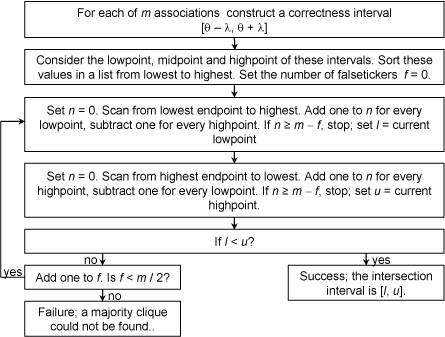Figure 2. Clock Select Algorithm

The algorithm operates as shown in Figure 2. Let m be the number of candidates and f the number of falsetickers, initially zero. Move a pointer from the leftmost endpoint towards the rightmost endpoint in Figure 1 and count the number of candidates, stopping when that number reaches mf; this is the left endpoint of the intersection interval. Then, do the same, but moving from the rightmost endpoint towards the leftmost endpoint; this is the right endpoint of the intersection interval. If the left endpoint is less than the right endpoint, the intersection interval has been found. Otherwise, increase f by 1. If f is less than n / 2, try again; otherwise, the algorithm fails and no truechimers could be found.

The clock select algorithm again scans the correctness intervals. If the right endpoint of the correctness interval for a candidate is greater than the left endpoint of the intersection interval, or if the left endpoint of the correctness interval is less than the right endpoint of the intersection interval, the candidate is a truechimer; otherwise, it is a falseticker.

In practice, with fast LANs and modern computers, the correctness interval can be quite small, especially when the candidates are multiple reference clocks. In such cases the intersection interval might be empty, due to insignificant differences in the reference clock offsets. To avoid this, the size of the correctness interval is padded to the value of `mindist`, with default 1 ms. This value can be changed using the `mindist` option of the `tos` command.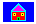Home Page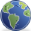Site Map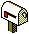Contacts